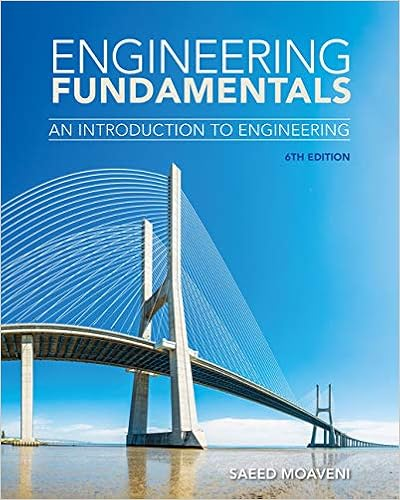# hw6ANS - Math 10 Homework 6 Answers to homework 1 What are...

• Homework Help
• 11
• 94% (70) 66 out of 70 people found this document helpful

This preview shows page 1 - 3 out of 11 pages.

##### We have textbook solutions for you!
The document you are viewing contains questions related to this textbook.The document you are viewing contains questions related to this textbook.
Chapter 19 / Exercise 19.10
Engineering Fundamentals: An Introduction to Engineering
MoaveniExpert Verified
Math 10 - Homework 6 Answers to homework 1. What are the two types of hypotheses used in a hypothesis test? How are they related?
2. Describe the two types of error possible in a hypothesis test decision.
True or False? In Exercises 3-8, determine whether the statement is true of false. If it is false, rewrite it as a true statement.
4. A statistical hypothesis is a statement about a sample. False, it is a statement about a population parameter.
5. If you decide to reject the null hypothesis, you can support the alternative hypothesis. True
6. The level of significance is the maximum probability you allow for rejecting a null hypothesis when
7. A large P-value in a test will favor a rejection of the null hypothesis. False, a small p-value supports rejecting the null hypothesis.
8. If you want to support a claim, write it as your null hypothesis. False, to support a claim write it as the alternative hypothesis.
In Exercises 9-14, use the given statement to represent a claim. Write its complement and state which is Ho and which is Ha. 9. Ha: p >.65 Ho: p ζ 65 10. Ho: ζ 128 Ha: >128 11. Ha: 2 5 Ho: 2 = 5 12. Ho: =1.2 Ha: 1.2 13. Ho: p η 0.45 Ha: p < 0.45 14. Ha: < 0.21 Ho: η 0.21
##### We have textbook solutions for you!
The document you are viewing contains questions related to this textbook.The document you are viewing contains questions related to this textbook.
Chapter 19 / Exercise 19.10
Engineering Fundamentals: An Introduction to Engineering
MoaveniExpert Verified
Think about the context of the claim. Determine whether you want to support or reject the claim. a. State the null and alternative hypotheses in words. b. Write the null and alternative hypotheses in appropriate symbols c. Describe in words Type I error (the consequence of rejecting a true null hypothesis.) d. Describe in words Type II error (the consequence of failing to reject a false null hypothesis.) 15. You represent a chemical company that is being sued for paint damage to automobiles. You want to support the claim that the mean repair cost per automobile is about \$650. How would you write the null and alternative hypotheses?
16. You are on a research team that is investigating the mean temperature of adult humans. The commonly accepted claim is that the mean temperature is about 98.6°F. You want to show that this claim is false. How would you write the null and alternative hypotheses?=
•••